You can split your deck of fact family cards into two piles: facts you are learning and facts you know. Use the activities to build concepts to help you learn and picture new facts. Use the activities to build speed to get faster with the facts you already know.

* activities to build concepts
Snap Them Together... Pull Them Apart
Draw the Family
Math on a Plate
Number Line Math
Build on What You Know

These activities will help you to picture each fact. Take as much time as you like with each fact family card.

* Snap Them Together... Pull Them Apart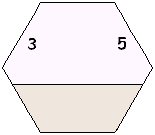You may use the cards without the "house" or place the cards in the "house" so that the bottom number is hidden. You will need plastic blocks or beads which snap together, such as: Unifix cubes Legos (all the same shape) Duplo blocks (all the same shape) snap-together beads
1) Using the card above as an example, snap together 3 blocks of one color.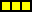2) Snap together 5 blocks of another color.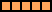3) Snap together the two sets of blocks and count them. There are 8.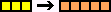4) Say or write the number sentence: 3 + 5 = 8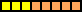5) Flip the stack of blocks over. Say or write the number sentence: 5 + 3 = 8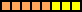6) Look at the whole card now to see the bottom number. Break the stack into two smaller stacks, one of each color. Hide one color. Say or write the number sentence: 8 - 3 = 5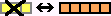7) Again snap the two stacks together and break them apart. Hide the other color. Say or write the number sentence: 8 - 5 = 3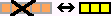8) Repeat steps 1 - 7 for each card in the "house."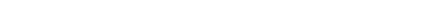up

* Draw the Family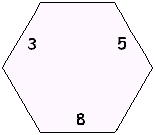Use the cards without the "house" so that you see all the numbers. You will need two colored pencils or markers. Make a simple drawing of each fact in the fact family. Write the fact under each picture.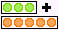3 + 5 = 8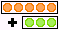5 + 3 = 8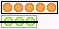8 - 3 = 5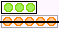8 - 5 = 3up

* Math on a Plate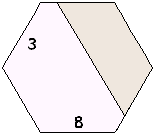Place the fact family "house" to cover one of the smaller numbers on the cards. You will need: * a plastic or paper plate divided into 3 sections * objects to count, such as:               dried beans               uncooked pasta               dry cereal, or               pebbles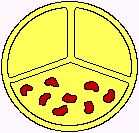1) Using the card above as an example, fill the large bottom section of the plate with 8 objects (to match the bottom number on the card).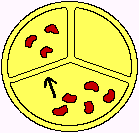2) Move 3 objects into one of the smaller sections.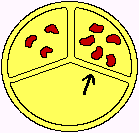3) Move the rest of the objects into the other small section. How many are there? That's the missing number hidden by the fact family "house."up

When subtracting from a number greater than 10, count up using a number ladder with 10 on the middle rung.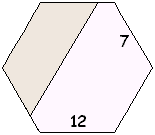Climb up the ladder:7 + 3 = 1010 + 2 = 12 You climbed 3 + 2, which is 5. 7 + 5 = 12(Can you think of how you might climb down the ladder and find a difference of 5?)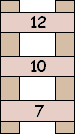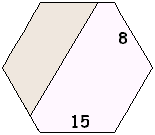Climb up the ladder:8 + 2 = 1010 + 5 = 15You climbed 2 + 5, which is 7. 8 + 7 = 15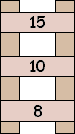up

* Number Line Math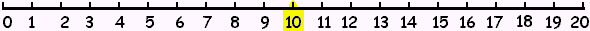Place the cards in the "house" so that one of the numbers is hidden. Several examples below show how to use the number line with different hidden numbers. You will need:
* a number line such as the one above or a ruler, with the 10 highlighted
* a few small triangles or other objects to place along the number line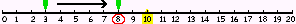3 + ? = 8Place a marker on the 3. Count up 5 more. What number do you land on? 8 is the hidden number. There are two ways to set up this card on the number line to find the hidden number.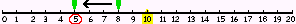8 - 3 = ?Place a marker on 8, the starting point. Count backward 3. Place a second marker where you land; that is the missing number.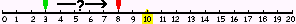3 + ? = 8Place a marker on 3 and another marker on 8. 3 plus (how many more) equals 8? (Using markers of 2 different colors may remind you that you are not adding these two numbers together but are counting from one number up to the second number.) In this example, you count forward 5 to reach the 8. 5 is the hidden number. When the sum is greater than 10, use 10 as an "anchor." The next examples show you how.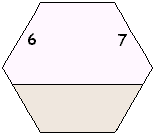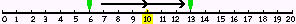6 + 7 = ?Place a marker on the 6. You need to move forward 7 more. Instead of counting up 7, break the 7 into two pieces (4 + 3) so you can first "hop" to the 10 and then hop the rest of the number. 6 + 4 = 10    ...   10 + 3 = 13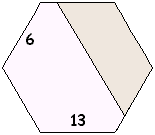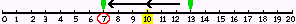13 - 6 = ?Place a marker on 13. You need to move backward 6 and see where you land. Instead of counting back 6 spaces, one at a time, break the 6 into two "hops" (3 + 3) so you can hop first to the 10.13 - 3 = 10    ...   10 - 3 = 7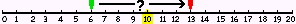6 + ? = 13Instead of counting one by one to move from 6 to 13, first "hop" 4 to reach 10.6 + 4 = 10 ... 10 + 3 = 13 Combine the two hops, 4 + 3, to get 7. 6 + 7 = 13
The more you add and subtract with a number line, the more you will start to form a "mental" number line in your mind which will help you later on to add and subtract much larger numbers.up

* Build On What You Know

Doubles + 1:
If you know your doubles, use them to figure out other facts.
6 + 6 = 12 so 6 + 7 = one more than 12

Flexible Fact Family:
2 + 9 = ?   It might be easier to add 9 + 2.

Instead of subtracting a large number, count up from that number:
12 - 9 = ?   becomes 9 + ? = 12 ... count up 3 to reach 12.

9 + 8 = ?    Think: 10 + 8 = 18, so 9 + 8 is one less, or 17.
7 + 8 = ?    Think: 7 + 10 = 17, so 7 + 8 is two less, or 15.

Use 10 as an Anchor as shown in the Number Line and Make a Ladder activities, above.up

* activities to build speed
These activities are meant to be used only after you have become very familiar with each card, using some of the ideas in the activities to build concepts section above.
Shrinking Time Limit
Hide Two
3-Minute Sort
Four Facts in the Family

* Shrinking Time Limit

Ask a partner to time you as you answer each card. Agree on a time limit. You might start with 10 seconds per card. If you answer a card correctly within the time limit, set it aside and go on to the next card. If you cannot answer the card correctly within the time limit, take all the time you need to figure it out, using objects or an idea from the Build On What You Know section above. Then explain out loud how you solved the problem. Set these harder cards in a different pile as you answer each one.

After you finish going through the stack, return to the pile of harder cards (which took you longer to answer). Repeat the steps above with the same time limit, seeing which harder cards you can now answer within the time limit, and taking as much time as you need to figure out the others and explain how you figured them out.

When you are able to go through the whole pile within your time limit, set a shorter time limit and repeat the steps above. Eventually your goal is to answer each card in 3 seconds or less!up

* Hide Two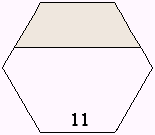2 + 9 3 + 84 + 7 5 + 6 Set the roof on your "house" so that the top two numbers of each card are hidden. Have a partner time you for 10 seconds while you say or write the pairs of numbers which might be hidden. Remember that the top two numbers are always single digits. The possible hidden pairs for this card are listed below it. (In this activity, the order of the two numbers doesn't matter. If you say "9 + 2" and the card has 2 on the left and 9 on the right, that is still fine. So you only need to mention each combination of numbers once.)
When the 10 seconds are up, look at the whole card. If you had said or written the pair of missing numbers (in either order), you keep the card. Otherwise, your partner keeps the card. Now it is your partner's turn to guess the missing numbers on the next card while you do the timing.

This game is partly skill and partly luck. But the better you know your facts, the faster you'll be able to say all of the possible pairs of hidden numbers, and you'll have a better chance of keeping your cards.up

* 3-Minute Sort
Place the cards in the fact family house, covering any of the 3 numbers on the cards. Use a 3-minute timer and see how many cards you can get through in three minutes, saying the missing number on each card before you lift it from the house. If you are correct, place the card in one pile. If you are wrong or can't answer, place the card in another pile.

When the three minutes are up, count how many cards are in each pile. Now place the fact family house so that it covers a different portion of each card, and time yourself again. Can you get through more cards in the stack this time?up

* Four Facts in the Family
For this activity, you don't need the fact family house. Look at each card and write the four facts in that fact family. (You can draw a house for each set of facts and write the facts inside of it if you like.)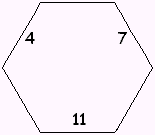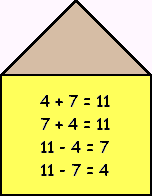You can do this as an untimed activity or see how many cards you can get through in a certain time.

how to use the + - cards

print the + - cards

multiplication / division cards